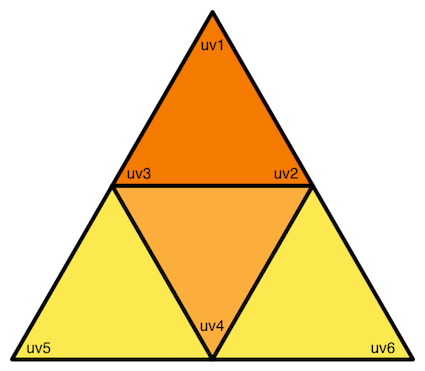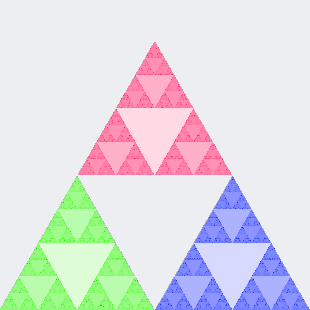I’ve been on a bit of a break from my normal jovial self due to a shit storm of bad stuff happening that I wont go into here, but hopefully this years going to be awesome. Anyway, here’s the next exciting installment in my series on MonoGame. (Well I find it exciting anyway :-) )

If you remember back to the last post I mentioned the platonic solids, and we created and rendered the tetrahedron, lets recap on what the five solids are:

1. Tetrahedron (four faces)
2. Cube or hexahedron (six faces)
3. Octahedron (eight faces)
4. Dodecahedron (twelve faces)
5. Icosahedron (twenty faces)

We covered the tetrahedron in the previous post and the hexahedron is pretty humdrum so I’m not going to cover that here so lets move onto the next one the octahedron.

## Creating the Octahedron

Here’s a function that we will use to generate an octahedron:

``````module Platonic
let createOctahedron()=
let top = Vector3.Up
let midOne =   top |> Vector3.transform (Matrix.CreateRotationX(toRad 90.0f) * Matrix.CreateRotationY(toRad 45.f))
let midTwo =   top |> Vector3.transform (Matrix.CreateRotationX(toRad 90.0f) * Matrix.CreateRotationY(toRad 135.f))
let midThree = top |> Vector3.transform (Matrix.CreateRotationX(toRad 90.0f) * Matrix.CreateRotationY(toRad 225.f))
let midFour =  top |> Vector3.transform (Matrix.CreateRotationX(toRad 90.0f) * Matrix.CreateRotationY(toRad 315.f))
let bottom =   top |> Vector3.transform (Matrix.CreateRotationX(toRad 180.f))

[| midOne; top;  midTwo
midTwo; top; midThree
midThree; top; midFour
midFour;  top; midOne
midOne; midTwo; bottom
midTwo; midThree; bottom
midThree; midFour; bottom
midFour; midOne; bottom |]
``````

You can see that the bulk of the code is centred around rotating a Y axis unit vector `top` around the X and Y axis. All the vertices around the centre od the octahedron lie on the same plain and are simply rotated by 90 degrees in the X axis and then rotated by multiples of 90 degrees in the Y axis starting at 45 degrees (45, 135, 225, 315). Finally the the `top` unit vector is flipped to the bottom by rotating around 180 degrees in the X axis, this forms the bottom point. The final step consists of combining the vertices into an array with the array syntax `[| ... |]` specifing each triangle of the octahedron in turn.

If you were looking carefully you might have noticed that the `Vector3.transform` function is not part of the MonoGame library. I wrapped MonoGames’s `Vector3.Transform` function so that the `Vector3` is the last parameter so we can use the forward pipeline operator `|>`:

``````module Vector3 =
let transform (m:Matrix) v =
Vector3.Transform(v, m)
``````

## Drawing the Octahedron

What now? Well, with this code we have just been working with the raw vertices, we now need to get this into a form that MonoGame can render, namely an array of the `VertexPositionColor` structure. It’s a bit of a mouthful so lets alias this so we can simply refer to it as `vpc`:

``````let vpc v c = VertexPositionColor(v, c)
``````

To render the octahedron we can now modify the draw method of the tetrahedron code from the last post maybe something like this should illustrate:

``````override x.Draw (gameTime) =
// Clear the backbuffer
x.GraphicsDevice.Clear (Color.CornflowerBlue)
for pass in basicEffect.CurrentTechnique.Passes do
pass.Apply()
let octahedron =
Platonic.createOctahedron()
|> Array.mapi (fun i -> Platonic.vpc (if i % 2 = 0 then Color.BlueViolet
else Color.Orange) )
x.GraphicsDevice.DrawUserPrimitives(PrimitiveType.TriangleList, octahedron, 0, octahedron.Length / 3)
``````

Bear in mind we are not looking at optimisation at all at this stage purely visualising what we have. We are using the `mapi` function to alternate between defining blue violet and orange vertex colours. At the moment because we haven’t set up any lights the octahedron would just appear as diamond chunk of colour with no shading, with these two simple vertex colours we can see the separate facets and see the 3D form.

## Subdivision Surfaces

According to Wikipedia:

A subdivision surface, in the field of 3D computer graphics, is a method of representing a smooth surface via the specification of a coarser piecewise linear polygon mesh. The smooth surface can be calculated from the coarse mesh as the limit of a recursive process of subdividing each polygonal face into smaller faces that better approximate the smooth surface.

They are also known as scalable geometry. I’m not going to get into the realm of true sub-divisional modelling such as providing a visible control surface with editing and crease support, I just wouldn’t be able to do it justice within the scope of this introductory series. Applications like Softimage or Maya are masters of sub-divisional modelling, you might want to check those out if you are interested in what can be done in that area. Subdivision surfaces have been quite popular in the computer graphics industry as it allows modellers and animators to work with simple mesh surfaces with far less control points that can be rendered with super smooth detail but without the constraints of having to work with millions of points on the screen at once which can be computationally very expensive and distracting. Nowadays that kind of processing is done by a GPU’s vertex shader’s or more recently the geometry shader’s which can take a simple triangle as an input and produce zero or more triangles as its output.

One of the properties of platonic solids is that all of the defining vertices lie on a sphere. If we were to take each of the defining faces or triangles and recursively divide them into four smaller triangles, and project each of the containing vertices onto the sphere then eventually we would get an approximation of a sphere. This was the basis of Charles Loop’s thesis Smooth Subdivision Surfaces Based on Triangles. What I am going to present here will not go into that level of detail and we will not be generating any control surfaces to act on the subdivision mesh. We could call this a poor man’s subdivision surface or sphere approximation :-).

Lets create a quick and dirty function to try this out anyway:

``````let rec subdivide(v1, v2, v3, depth) =
seq{match depth with
| 0 -> yield vpc Color.LightBlue (v1 |> Vector3.Normalize)
yield vpc Color.AliceBlue (v2 |> Vector3.Normalize)
yield vpc Color.SlateGray (v3 |> Vector3.Normalize)

| _ -> let u12 = ((v1 + v2) / 2.0f) |> Vector3.Normalize
let u23 = ((v2 + v3) / 2.0f) |> Vector3.Normalize
let u31 = ((v3 + v1) / 2.0f) |> Vector3.Normalize

yield! subdivide(v1, u12, u31, depth-1)
yield! subdivide(v2, u23, u12, depth-1)
yield! subdivide(v3, u31, u23, depth-1)
yield! subdivide(u12, u23, u31, depth-1) }
``````

Here we have a recursive function that takes three vertices `v1, v2, v3` and a depth parameter. When the depth parameter is zero we are at our subdivision maximum and we return a normalized triangle. Incidentally for the same lighting issues mentioned above we use three different colours for the vertices: light blue, alice blue, and slate grey. The three vertices `u12, u23, u31` define the points in-between the input triangle, we calculate them by adding the vertices together and dividing them by two `((v1 + v2) / 2.0f)` then pipe-lining the result to the normalize function (`|> Vector3.Normalize`). We do this for each of the points. The final step is the `yield!` section which creates the next level of subdivision for each of the resulting four triangles. Remember our input triangle is divided into four. If fact in the previous article there are several images of this:Tetrahedron-coordinatesTetrahedron

The Sierpinski triangle (without the holes) is actually our subdivision method, except the we subdivide every triangle produced.

To try this out lets change the `Draw` method so that it looks like this:

``````override x.Draw (gameTime) =
x.GraphicsDevice.Clear (Color.CornflowerBlue)
for pass in basicEffect.CurrentTechnique.Passes do
pass.Apply()
let subdiv = Platonic.createOctahedron()
|> Seq.windowed 3
|> Seq.map (function
| [|a;b;c|] -> subdivide(a,b,c, 3)
| _ -> failwith "Unsupported array size." )
|> Seq.concat
|> Seq.toArray
x.GraphicsDevice.DrawUserPrimitives(PrimitiveType.TriangleList, subdiv, 0, subdiv.Length / 3)
``````

Here we are using some of the functions from the sequence module to group and process the vertices.

• First the result of `Platonic.createOctahedron()` is grouped into triangles using `Seq.windowed 3`.
• Now we map each the triangle using the using the `subdivide` function.
• Next we merge the sequence back together using `Seq.concat`.
• Finally we convert the sequence back into an array with `Seq.toArray`.

The image below shows the octahedron at various levels of subdivision from one through to four:

Well I hope you enjoyed this brief sojourn into subdivision, if you want to investigate further I recommend looking at the following papers.

It’s a very interesting area and I dont think will be able to resist doing another article delving deaper later on.

Until next time…